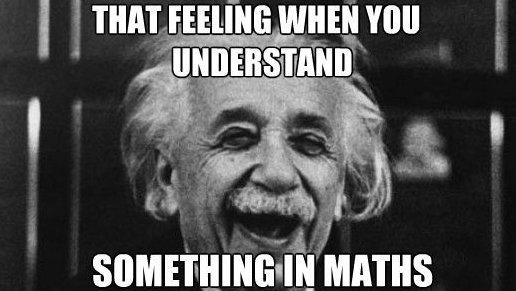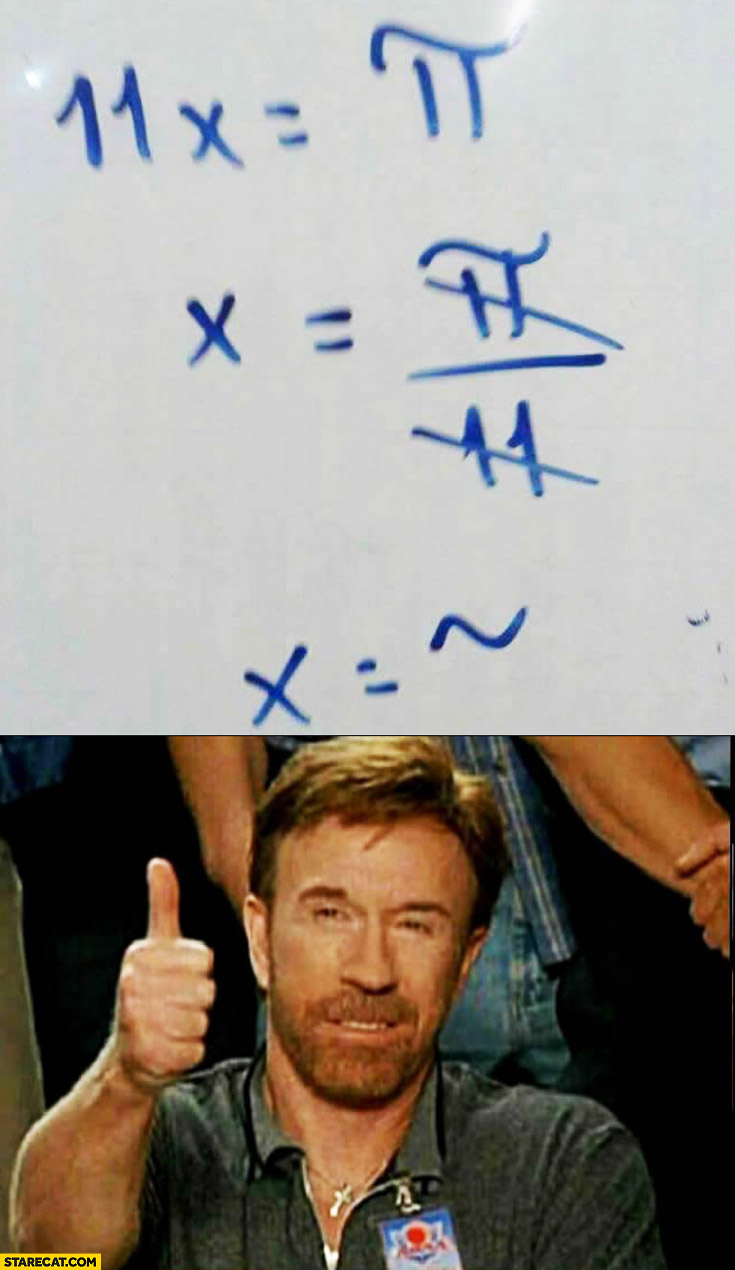# Mates y TIC - Maths and ICT

## Actividades de Matemáticas con TIC - Math Activities with ICT - - - (matesytic@gmail.com) Ricardo García Mesa

•# Archive for November, 2018

## Nonlinear systems of equations

Posted by ricardogm on 28th November 2018Hi

As you probably remember, last year we learnt to solve sytems of linear equations (first degree equations). Now we are going to try something a bit more difficult: systems of nolinear equations. In these systems, one or more of the equations are at least of second degree  and/or there is a product of variables in one of the equations.. The method we are going to use is substitution, because it’s the easiest, and in many cases the only practical way to solve these problems. Sometimes elimination works as well, or equalization. The graphic method is very helpful here, if you have access to geogebra, for instance. (HINT: I bet you have, it works on your phone! →Download page)

Here you can find some solved examples.

Here is a video showing one example. The audio is fine, but if you don’t have headphones the images are clear enough:

A written explanation is given in this book, page 31. Read it:

Book 4º ESO

Now some practice: Download these exercises and try to solve, in your notebook, as many as posible. The solutions are at the end, check them.

Another way to solve these or other systems is graphically. We are going to use Geogebra with the exercises. The teacher is going to show you how.Posted in 4º ESO | No Comments »

## Factoring polynomials

Posted by ricardogm on 22nd November 2018Hi

Now you have several tools to factorize polynomials (common factor, Ruffini, second degree formula).

For example, a second degree polynomial can be factorized using the formula, and writing it this way:

a x2 + bx + c =a · (x − s1) · (x − s2

being s1, s2, the solutions or roots of the polynomial.

An important word to use here is root. We say that x=a is a root or zero of the polynomial P(x) if P(a)=0. That means that this same x=a is a solution of the equation P(x)=0

With that in mind, you can use the method we saw for biquadratic equations as well. Use it to find the solutions (=roots) and then you can write the factorization this way:

ax4 + bx2 + c =a·(x-s1)(x-s2)(x-s3)(x-s4)

being s1, s2, s3, s4 the solutions or roots of the polynomial,Now some exercises to work out in your notebook. Use Symbolab to check them.

#### Factor and Calculate the Roots of the Following Polynomials

a) x3 + x2

b) 2x4 + 4x2

c) x2 − 4

d) x4 − 16

e)  9 + 6x + x2

f)g) x4 − 10x2 + 9

h) x4 − 2x2 − 3

i) 2x4 + x3 − 8x2 − x + 6

Now solve these equations. You should use the same tools, the only difference is the solution you have to give me.

a) 2x3 − 7x2 + 8x − 3

b) x3 − x2 − 4

c) x3 + 3x2 − 4 x − 12

d) 6x3 + 7x2 − 9x + 2

e) 3x5 − 18x3 + 27x =

f) 2x3 − 50x =

g) 2x5 − 32x =

h) 2x2 + x − 28 =Posted in 4º ESO | No Comments »

## Soluciones temas 1, 2 y 3

Posted by ricardogm on 22nd November 2018Muy buenas

Matrices

Determinantes

Sistemas

Y los de PAU-EBAU

En UNIOVI también tienen con corrección los de EBAU

Posted in 2º Bach. CT | No Comments »

Posted by ricardogm on 15th November 2018

Muy buenas

Os enlazo aquí un blog muy completo sobre el tema:

Unas demostraciones gráficas de las leyes de De Morgan:

Diagramas de Venn

Y unas herramientas:

Posted in 2º Bach. CT | No Comments »

## The All Mighty Zero Product Property

Posted by ricardogm on 12th November 2018That’s it. The Zero Product Property simply states that if ab=0 , then either a=0  or  b=0 (or both). A product of factors is zero if and only if one or more of the factors is zero. Pretty logical, huh?

As simple as it seems, it’s so powerful that we can solve polynomial equations of any degree using this method,  if we can factor the polynomial (using Ruffini, for example).Here, we have found, for example, that 1 and -2 are solutions ofThere’s one solution more that we could find with Ruffini. Can you find it?

It also explains the relationship between factors of a polynomial and x-intercept of a function:

For example, if we plot this polynomial as a function:We get this:And you can see that the function crosses the x-axis on x=1 and x=-2 (and in x=-1, because we could also get this with ruffini)

All in all, it´s clear that zero is a very interesting number (although very feared by students all over the world).

A very complete (and lenghty) summary of this can be found here.

Some exercises to practice:

Exercises

And some more.Posted in 4º ESO | No Comments »

## Equations review

Posted by ricardogm on 8th November 2018Hi

1. First of all, 5 minutes to check the equations from yesterday using this page of Symbolab.

2. Now solve as many of these equations as you can in a blank sheet of paper (to give to the teacher at the end of the class, with your name on it) and check the solutions with Symbolab.

a) x4 − 10x2 + 9 = 0

b)c) x4 − 61x2 + 900 = 0

d)e)f)g) Two natural numbers differ by two units and the sum of their squares is 580. What are these numbers?

h) A rectangular garden 50 m long and 34 m wide is surrounded by a uniform dirt road. Find the width of the road if the total area of the garden and road is 540 m².Posted in 4º ESO | No Comments »